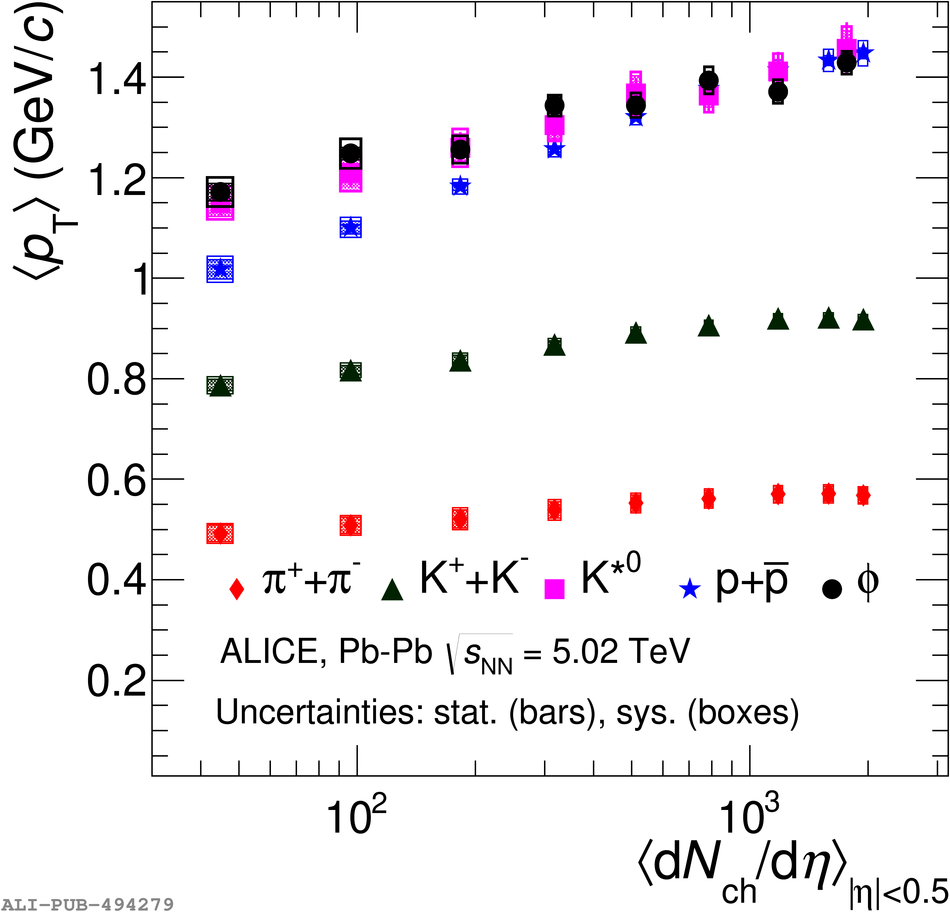# Figure 10

 Mean transverse momentum of \kstar and \phim as a function of the averaged charged particle density (\avdndeta$_{|\eta|\textless0.5}$) in \PbPb collisions at \snn $=$ 5.02 \TeV compared with the corresponding values for other identified particles such as $\pi^{\pm}$, K$^{\pm}$, and p($\bar {\rm p}$)~. The statistical uncertainties on the data are shown by bars and the systematic uncertainties by boxes. The shaded boxes show the un-correlated systematic uncertainties.